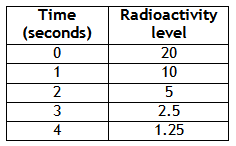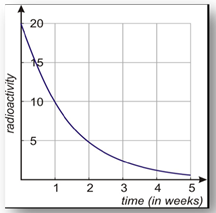Application

Exponential decay problems appear in several application problems. Some examples of these are half-life problems, and depreciation problems.

Example 1 A radioactive substance has a half-life of one week. In other words, at the end of every week the level of radioactivity is half of its value at the beginning of the week. The initial level of radioactivity is 20 counts per second.

a) Draw the graph of the amount of radioactivity versus the time in weeks.
b) Find the formula that gives the radioactivity in terms of time.
c) Find the radioactivity left after three weeks

Step 1. Make a table of values to represent the function.Step 2. Plot the points to make a graph.